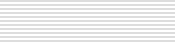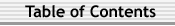Math 306 Homework from Linear Algebra, by Petersen Chapter 1 1.4: 1-8 Due 9/27 - Problems 1,5 are to be carefully written up and separated from the rest of the HW. for 6, the line over γ means complex conjugate: If γ=a+bi, then conj(γ)=a-bi. The key properties of the conjugate that need verification are that conj(γ1γ2)=conj(γ1)conj(γ2) and conj(γ1+γ2)=conj(γ1)+conj(γ2). Also if γ is real, note that conj(γ) = γ since the imaginary part of γ is 0. Solutions 1.5: 1-3,5-7,9-11 Due 10/4 for 2, p0≠ 0. for 8, identify xi with xi+ i0 = (xi,0). Remember the complexification uses complex scalars. for 10, replace start of hint with: "Assume A ε M is such that some entry is non-zero. Make it 1, by multiplying A on the left by an appropriate matrix. Then show ..." Consider the effect of left and right multiplication of a matrix A by a standard basis vector Eij described in example 10. Solutions 1.6: 2,4,5,6,8-10 Due 10/11 1.7: 1-3,7,9 1.8: 1-3,5,11,13 Due 10/18 Solutions 1.9: 1-4 1.10: 1-6 Due 10/29 Solutions (section 9) Solutions (section 10) 1.11: 1,4,6,8 1.12: 2,4,8,9,10 Due 11/8 Solutions (section 11) Solutions (section 12) Chapter 2 2.3: 1ab,2ab,3a,4ab,13,16,21 Due 11/16 Solutions 2.4: 1,4,6,7,8 Due 11/23 Solutions 2.5: 1ab,2ab,3a,4ab,8,13,15,18,19 Due 12/8 Most of the work for 1-4 was already done in section 3 HW Solutions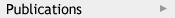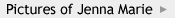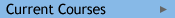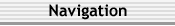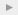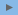SyllabusHomework